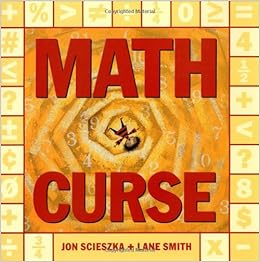page contents

UNIT 3 - Lesson 11 Video Links

Multiplication - Partial Products (4-digit x 1-digit)

Multiplication - Partial Products (2-digit x 2-digit)

Multiplication - Area Model (2-digit x 1 digit)

https://www.bing.com/videos/search?q=multiplication+area+model&&view=detail&mid=7EBBF673F2B968D471237EBBF673F2B968D47123&&FORM=VRDGAR

Multiplication - Area Model (3-digit x 1 digit)

https://www.bing.com/videos/search?q=multiplication+area+model&&view=detail&mid=5A8A145B3922D05AF8B65A8A145B3922D05AF8B6&&FORM=VRDGAR

Multiplication - Area Model (2-digit x 2-digit)

Click on the image to listen to Math CurseMath Playgroud

Math-Play

Check out the National Library of Virtual Manipulatives

Place Value Games:

Practice Saying REALLY big numbers

Ordering Numbers

Word-Form Mystery Picture

Place Value Puzzler

Football Place Value

Place Value Hockey

Place Value Game

Tank Place Value

practice comparing numbers

Place-Value Millionaire

BIG numbers Practice creating the largest numbers possible

Number Monster

Hoop Shoot

Four digit numbers

Addition Who wants to be a Millionaire?

Subtraction with regrouping- step by step game

Multiplication Games

Math Games to Practice Multiplication

Multiplication timed Challenge

Bracket Challenge

Fun Math Fact Practice

Open Arrays

Multiplication Blocks

Open Arrays Interactive Whiteboard

Division Games

Division Fruit Shoot

Demolition Division

Division Games

Sum Sense Division

Long Division:

Help Snork Divide!

Long Division with instructions

Dividerama

Long Division with remainders practice

Long Division How-to Video

Story Problems:

Practice solving story problems using a bar diagram

Fractions:

Fraction Models

Prime Number Fruit SPLAT

Simple Fraction Matching

Mixed Numbers Matching

Equivalent Fractions

Compare Fractions Balloon Pop

Comparing Fractions

Fraction SPLAT

PAC-MAN Equivelant Fractions

LCD

GCF

Reduce to Lowest Terms

Simplest Form Soccer Game